# Scikit学习–使用Python进行机器学习

二维码 73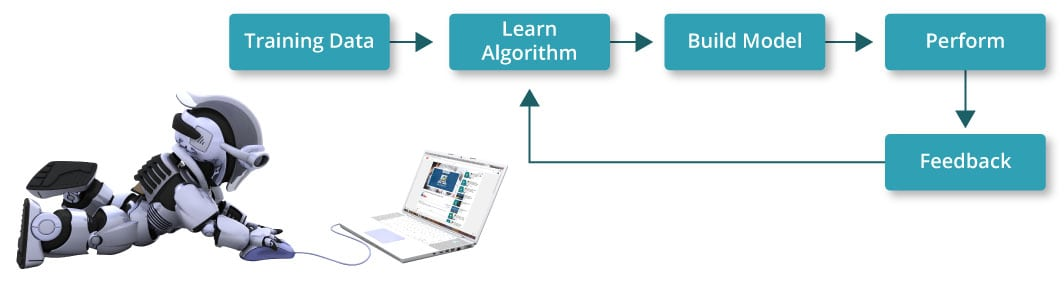Scikit Learn概述

Scikit learning是用于在Python中执行机器学习的库。 Scikit Learn是一个开放源代码库，已获得BSD许可，并且可以在各种情况下重用，鼓励学术和商业用途。 它在Python中提供了一系列有监督和无监督的学习算法。 Scikit学习包括流行的算法和库。 除此之外，它还包含以下软件包：

• NumPy
• Matplotlib
• SciPy (Scientific Python)

pip install scipy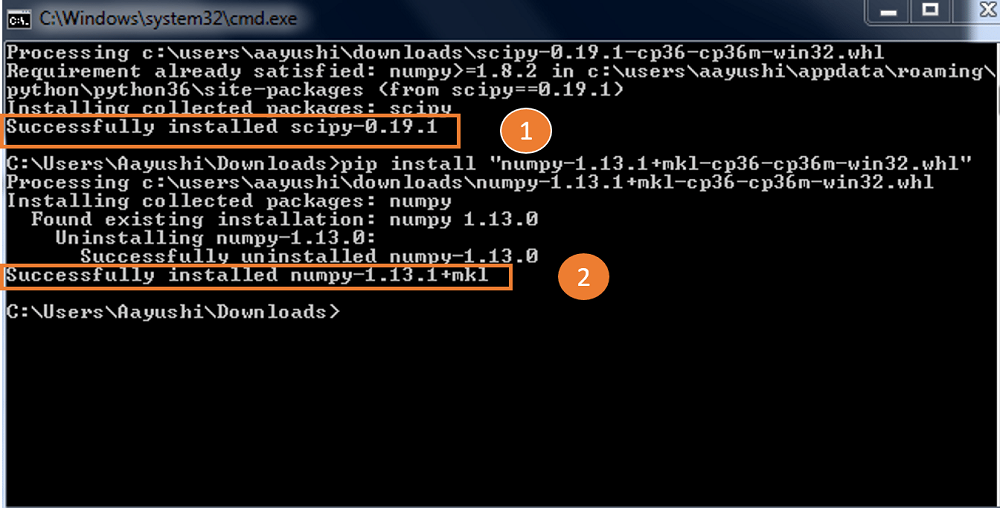Scikit learning提供了示例数据集，例如iris和digits。 您可以导入数据集并使用它们。 之后，您必须导入SVM，它代表支持向量机。 SVM是机器学习的一种形式，用于分析数据。

 import matplotlib.pyplot as pltfrom sklearn import datasetsfrom sklearn import svm digits= datasets.load_digits()print(digits.data)

 [[ 0. 0. 5. ..., 0. 0. 0.] [ 0. 0. 0. ..., 10. 0. 0.] [ 0. 0. 0. ..., 16. 9. 0.] ..., [ 0. 0. 1. ..., 6. 0. 0.] [ 0. 0. 2. ..., 12. 0. 0.] [ 0. 0. 10. ..., 12. 1. 0.]]

 import matplotlib.pyplot as pltfrom sklearn import datasetsfrom sklearn import svm digits= datasets.load_digits()print(digits.target)print(digits.images)

 [0 1 2 ..., 8 9 8]                  // target of the data[[ 0. 0. 5. 13. 9. 1. 0. 0.]         // image of the data [ 0. 0. 13. 15. 10. 15. 5. 0.] [ 0. 3. 15. 2. 0. 11. 8. 0.] [ 0. 4. 12. 0. 0. 8. 8. 0.] [ 0. 5. 8. 0. 0. 9. 8. 0.] [ 0. 4. 11. 0. 1. 12. 7. 0.] [ 0. 2. 14. 5. 10. 12. 0. 0.] [ 0. 0. 6. 13. 10. 0. 0. 0.]]

 import matplotlib.pyplot as pltfrom sklearn import datasetsfrom sklearn import svm digits= datasets.load_digits()                     // datasetclf = svm.SVC(gamma=0.001, C=100)print(len(digits.data))x,y=digits.data[:-1],digits.target[:-1]            // train the dataclf.fit(x,y)print('Prediction:', clf.predict(digits.data[-1])) //predict the dataplt.imshow(digits.images[-1],cmap=plt.cm.gray_r, interpolation="nearest")plt.show()

1796

Prediction: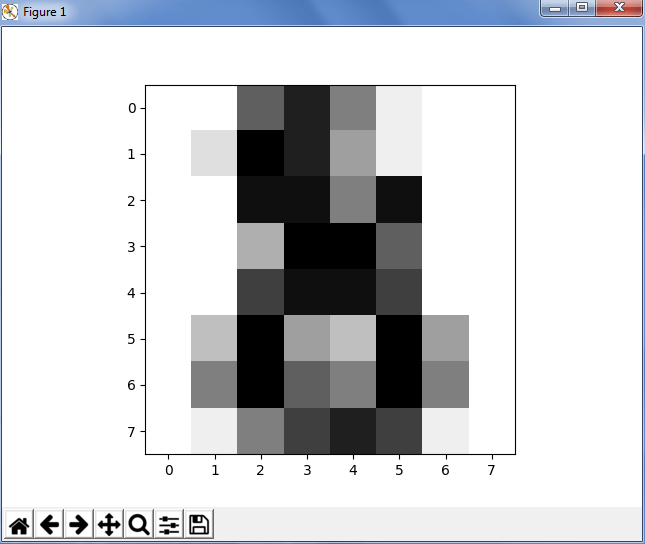import matplotlib.pyplot as pltfrom sklearn import datasetsfrom sklearn import svm digits= datasets.load_digits()# Join the images and target labels in a listimages_and_labels = list(zip(digits.images, digits.target)) # for every element in the listfor index, (image, label) in enumerate(images_and_labels[:8]):    # initialize a subplot of 2X4 at the i+1-th position    plt.subplot(2, 4, index + 1)    # Display images in all subplots    plt.imshow(image, cmap=plt.cm.gray_r,interpolation='nearest')    # Add a title to each subplot    plt.title('Training: ' + str(label)) # Show the plotplt.show()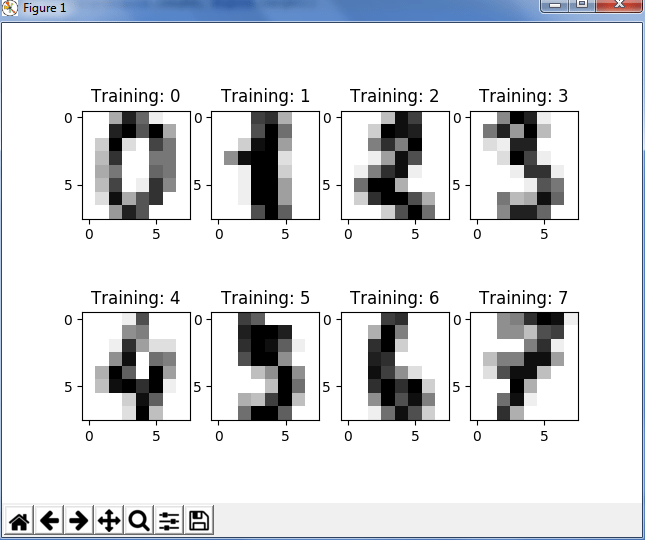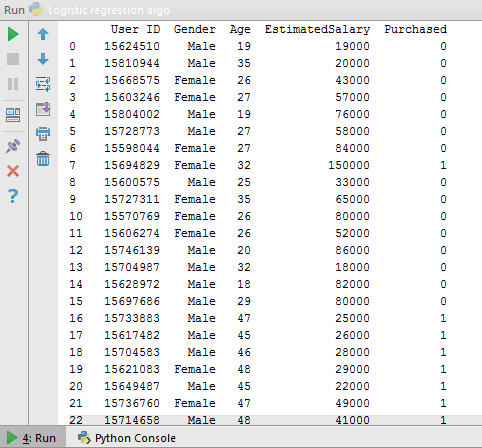• 是和否
• 真和假
• 高和低
• 通过和失败

Settings -> Add Package ->   Pandas -> Install

 from sklearn.cross_validation import train_test_split X_train, X_test, y_train, y_test = train_test_split(X, y, test_size = 0.25, random_state = 0)

 from sklearn.preprocessing import StandardScaler sc = StandardScaler() X_train = sc.fit_transform(X_train) X_test = sc.transform(X_test)

 from sklearn.linear_model import LogisticRegression classifier = LogisticRegression(random_state = 0) classifier.fit(X_train, y_train)

 y_pred = classifier.predict(X_test)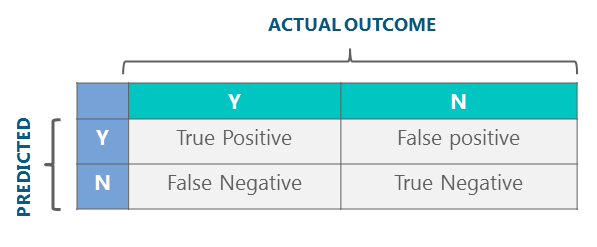from sklearn.metrics import confusion_matrix cm = confusion_matrix(y_test, y_pred) print(cm)

 [[65 3] [ 8 24]]

= TP + TN / FN + FP

= 65+24 / 65 +3+ 8 + 24

=89%

 from sklearn.metrics import accuracy_score    // import the function accuracy_scoreprint(accuracy_score(y_test, y_pred)*100)     // prints the accuracy

89.0